Berg, S., Turesson, J., Ekevad, M., and Björnfot, A. (2019). "In-plane shear modulus of cross-laminated timber by diagonal compression test," BioRes. 14(3), 5559-5572.

#### Abstract

Cross-laminated timber (CLT) is an engineered wood material that is used in the construction industry, e.g., for floors, walls, and beams. In cases where CLT-elements are used as shear walls, the in-plane-stiffness is an important property. For non-edge glued CLT, in-plane shear stiffness is lower than for edge-glued CLT. To evaluate the non-edge glued CLT panel’s in-plane shear modulus, the diagonal compression test and finite element (FE) simulation was used. FE-models with both isotropic and orthotropic material models were used to calculate the shear stiffness. The FE models using pure shear loads were used as a reference to determine the correct value of the shear modulus. To verify the FE simulations, diagonal compression tests were conducted on 30 CLT samples. A calibration formula was derived using the least square method for calculation of shear modulus. The formula gave accurate results. The results showed that FE simulations can reproduce the same shear stiffness as tests of non-edge glued 3-layer and 5-layer CLT panels.

In-plane Shear Modulus of Cross-laminated Timber by Diagonal Compression Test

Sven Berg,a,* Jonas Turesson,a Mats Ekevad,a and Anders Björnfot b

Cross-laminated timber (CLT) is an engineered wood material that is used in the construction industry, e.g., for floors, walls, and beams. In cases where CLT-elements are used as shear walls, the in-plane-stiffness is an important property. For non-edge glued CLT, in-plane shear stiffness is lower than for edge-glued CLT. To evaluate the non-edge glued CLT panel’s in-plane shear modulus, the diagonal compression test and finite element (FE) simulation was used. FE-models with both isotropic and orthotropic material models were used to calculate the shear stiffness. The FE models using pure shear loads were used as a reference to determine the correct value of the shear modulus. To verify the FE simulations, diagonal compression tests were conducted on 30 CLT samples. A calibration formula was derived using the least square method for calculation of shear modulus. The formula gave accurate results. The results showed that FE simulations can reproduce the same shear stiffness as tests of non-edge glued 3-layer and 5-layer CLT panels.

Keywords: Cross-laminated timber; Finite element analysis; In-plane shear stiffness; Diagonal compression test; Shear modulus

Contact information: a: Department of Engineering Sciences and Mathematics, Division of Wood Science and Engineering, Skellefteå Campus, Luleå University of Technology, Forskargatan 1, Skellefteå SE-931 87, Sweden; b: Faculty of Engineering, Department of Manufacturing and Civil Engineering, Norwegian University of Science and Technology, Gjøvik, Norway; *Corresponding author: bersve@ltu.se

INTRODUCTION

Cross-laminated timber (CLT) is used frequently as an engineered wood product, especially for constructing multi-storey tall buildings. Common CLT-elements consist of at least three layers of boards where each board layer is adhesively bonded (glued) to the neighbouring layers and have alternating fibre directions. The side-by-side aligned boards in each layer can be glued or non-glued on their edges. In edge-glued CLT, each board layer forms a continuous plate, whereas in non-edge glued CLT, each layer consists of boards lying side by side but not connected to each other. CLT elements are mainly used to build up walls and floors. If used as shear walls in buildings, the in-plane shear stiffness is an important property (Lukacs et al.2019).

The properties of CLT, in general, depend on a multitude of parameters: the strength and stiffness of the input board material, the number and thickness of the layers, and the arrangement of the layers (Brandner et al. 2016). For edge-glued CLT panels, the in-plane shear stiffness is the same as the shear stiffness of solid wood, meaning for Norway spruce that it is on the order of about 700 MPa (Brandner et al. 2015), expressed as shear modulus. Franzoni et al. (2017) found that for non-edge glued CLT, the in-plane shear stiffness is substantially (30% to 50%) lower, 300 to 500 MPa, than for edge-glued CLT.

Several test methods have been used to measure and establish the properties of CLT-panels. Using a single node test for determining the properties of CLT-panels is not advisable (Brandner et al. 2018). However, there are a few different in-plane shear test methods for determining the properties of larger CLT-panels reported in the literature (Dujic et al. 2007; Andreolli et al.2014; Bjørnfot et al. 2017; Brandner et al. 2017).

Bjørnfot et al. (2017) performed the direct shear test, also called the picture frame test, as shown in Fig. 1(a). This test simultaneously compresses and stretches a square sample in the two diagonal directions. The direct shear test, using a steel frame to fix the test sample, is a common method to characterize the in-plane shear behaviour of textile reinforcements (Li et al.2014). According to Colman et al. (2014), the picture frame test allows application of biaxial pre-stress by clamping the shear specimen along its edges. This gives the specimen a uniform displacement resulting in a homogenous state of pure shear, which allows for measurement of the shear modulus.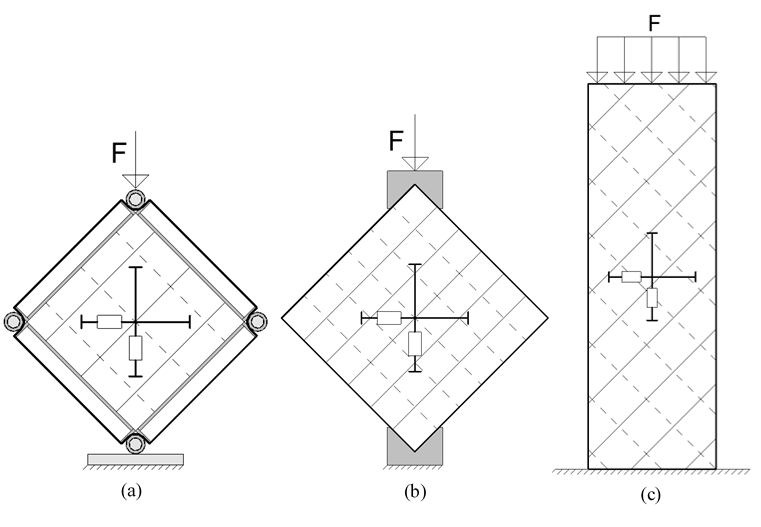Fig. 1. (a) Direct shear frame Bjørnfot et al. (2017), (b) diagonal compression test Dujic et al. (2007) and Andreolli et al. (2014), c) testing performed by Brandner et al. (2017). Displacement sensors are shown as lines and rectangles.

Dujic et al. (2007) tested three specimens of 3-layer CLT wall segments (30 × 30 × 9.4 cm in size) to obtain the shear modulus. This was done by compressing the specimens in the diagonal direction and measuring the vertical and horizontal displacements, as shown in Fig. 1(b). The mean values of the elastic shear modulus spanned from 500 to 650 MPa.

Brandner et al. (2017) used a CLT specimen with 1500 mm height and 500 mm width. These were cut at 45° from the main direction and compressed according to the approach proposed by Kreuzinger and Sieders (2013). The testing arrangement is illustrated in Fig. 1(c). In total, 112 mainly non-edge-glued specimens from three different manufacturers were investigated. The proposed values of the shear modulus for net-shear (non-edge glued) was 450 MPa, and for gross-shear (non-edge glued) 650 MPa.

Andreolli et al. (2014) used a diagonal compression test on CLTs to determine the elastic shear modulus. A total of four different panels were tested, one 3-layer panel and three 5-layer panels. One 3-layer and one 5-layer panel were edge glued; the others were not. All of the boards were of C24 strength class. The mean values of the elastic shear modulus spanned from 536 to 730 MPa, where 730 MPa was the value for one of the edge-glued CLT panels. A correction factor from Frocht (1931) was used to compensate for the deviation from pure shear stress that exists in this test procedure.

A state of pure shear is the goal in tests to calculate the shear modulus. However, none of the test methods described above will give a pure shear loading. The existing Frocht method to calculate correction factors during diagonal compression is based on isotropic behaviour, and it may not be suited for orthotropic materials such as the CLT-panel. Therefore another approach is needed for calculating correction factors for the diagonal test. Analytical or finite element (FE) models can be used to determine these correction factors.

This paper investigated and improved the ability of the diagonal compression test method to measure the shear stiffness of 3- and 5-layer non-edge glued CLT panels. Diagonal compression tests and FE simulations were conducted in parallel and compared. FE simulations using pure shear models were used as a reference to determinate the correct reference shear modulus. Appropriate correction factors were calculated using the least square method. These correction factors together with results from the diagonal compression tests gave reliable values for the shear modulus of CLT-panels.

EXPERIMENTAL

FE Simulations

Both isotropic and orthotropic material data were used in the FE simulations, as shown in Table 1. The orthotropic material ratio was obtained using the Swedish Standard SS-EN 338 (2016) for structural timber. Index l is the longitudinal fibre direction, and index r and t are the radial and tangential directions, respectively. 587 clear wood samples were taken from three of the 3-layer and from three of the 5-layer panels used in the diagonal compression test. El was measured by a 4-point-bending test according to Swedish standard SS-EN 408:2010+A1:2012 (2012). The same elastic modulus, Er, was used for both radial and tangential directions. The same shear modulus, Glt, was used for shearing along the surface normal to the longitudinal fibre direction. The third shear modulus was the rolling shear modulus, denoted Grt. All three independent Poisson’s ratios were set to zero according to Swedish standard SS-EN 1995-2 (2004), partly due to uncertainty about reliable data and their negligible effect on the result. A Cartesian coordinate system was used to specify orthotropic material directions.

For the isotropic wood material, Young’s modulus was arbitrarily set to 300 MPa, and Poisson’s ratio was set to 0.25. An isotropic material was chosen for a comparative analysis of a panel during pure shear stress and during a diagonal compression test. The isotropic material was used due to its known modulus of shear and, in this case, suitability to determine if the measured G value from the pure shear and diagonal compression tests was pure shear or not.

Table 1. Material Properties Used in the FE Simulations of Pure Fiber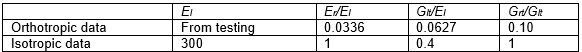FE simulations of pure shearShear l = Fibre, r = Radial and t = Tangential direction; Unit = MPa

Pure shear was defined as the case where uniform shear stress was applied on all four sides of a square CLT panel (Eq. 1),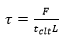(1)

where F is the shear force (N), L is the side length (m) and tclt is the total thickness (m) of the panels, as shown in Fig. 2. The 600 mm x 600 mm panels with board thicknesses/widths 29 mm/180 mm and 20 mm/156 mm for 3- and 5-layer panels, respectively, were studied. Assuming small displacements, the shear angle γ (rad) was calculated by Eq. 2,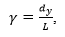(2)

where dy (m) is the displacement in the y-direction, illustrated in Fig. 2. The simulations were performed as linear elastic analyses and the shear modulus attained from the pure shear models; Gpure (Pa) was calculated according to Eq. 3,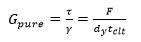(3)

All FE simulations were carried out using the ABAQUS 6.14-4 software (Dassault Simulia, Johnston, RI, USA), standard FE-code. The CLT panels were built up in three or five layers using individual board parts assembled to a panel. The C3D20R elements, a cuboid with 20 nodes and reduced integration, and a mesh size of 10 mm were used in all models. Glued contact surfaces were simulated using contact conditions which did not allow any movement between contact areas. This means that tangential slip was prohibited, and in the normal direction, a hard contact condition was used to keep the surfaces from penetrating each other. Free body movements were prevented by fixing two corner nodes, and a symmetry plane was introduced in the thickness direction of the panel. Corner point three was prevented from moving in the x- and y-directions. Corner two was prevented from moving in only the x-direction (Fig. 2).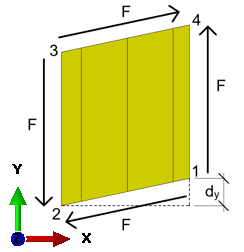Fig. 2. Pure shear model showing boundary conditions and applied forces. One corner point three is prevented from moving in the x- and y-directions and the point two was prevented from movement in the x-direction. The displacement, dy, was measured in the y-direction at point 1. Black arrows illustrate the surface traction force F

In total, eight FE simulations for the pure shear load case were conducted, as shown in Table 2. Both edge glued (Eg) and non-edge glued variants were studied. Isotropic (iso) and orthotropic material models were also studied.

Table 2. List of the Eight FE Simulated Pure Shear (Ps) Models and their Characteristics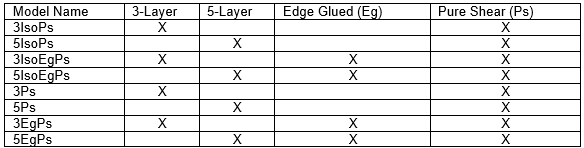Models with a name which includes “Iso” had isotropic material properties, all other models had orthotropic material properties.

FE simulations of diagonal compression

Twelve FE simulations of diagonal compression were conducted; six 3-layer and six 5-layer simulations (Table 3, Fig. 3). Some panels had holes and cut outs, because they already had been used in a picture frame test, to measure the shear modulus. Models with a name including “Iso” were constructed with isotropic material properties and models without had orthotropic material properties. “Eg” stands for edge glued. The same isotropic and orthotropic material data was used as in the pure shear simulations (Table 1).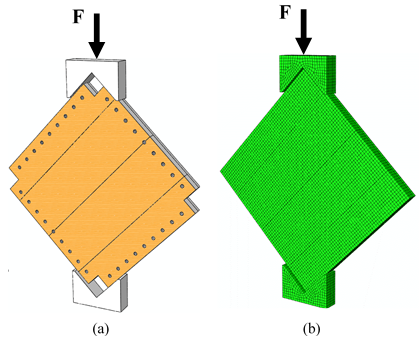Fig. 3. Diagonal compression model showing panel with holes (used in another study) and steel crotches to induce displacement (a) and a mesh panel without holes (b)

Table 3. List of the 12 FE Simulated Models and their Characteristics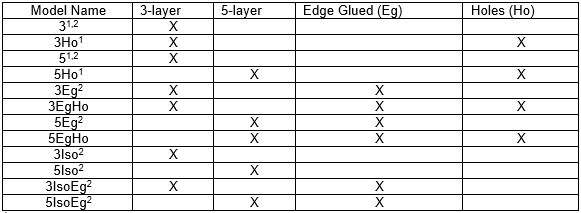1 Same as diagonally compression tested panels

Used for least square calibration

Models whose name includes “Iso” had isotropic material properties; all other models had orthotropic material properties. “Ho” stands for models with holes and cut-outs. “Eg” stands for edge glued and 3 and 5 stand for 3-layer and 5-layer panels, respectively.

In the FE simulations of diagonal compression, forces were applied as illustrated in Fig. 3, and the displacements were measured in the x- and y-direction using the “+” marks shown in Fig, 4. The measurement was done in the central region representing 16% of the total area of the panel as was initially proposed by Andreolli et al. (2014).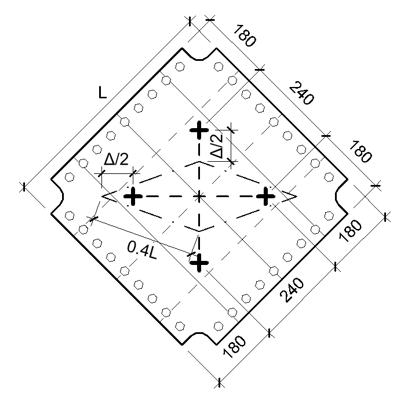Fig. 4. Panel with holes (Ho) showing the measuring points marked with +. Lengths for vertical (active) and horizontal (passive) displacement sensors are indicated with lines. The dash-dotted lines illustrate the central region after loading. Δ is the displacement of the sensors.

Calculation of shear modulus from diagonal compression FE simulations

From the FE simulated diagonal compression models described above, a method to calculate the shear modulus, G, was sought. The Gpure-values from the pure shear FE simulations, calculated as described above, were viewed as the correct value of G. To be able to calculate the G-value, the stiffness values from the FE simulations of diagonal compression were used to calculate a fictitious value, G* (Pa), in the following way:

First, the fictitious shear value, G*, was defined as the shear stress, τ, divided by the shear strain, γ. Shear stress and strain were constant in the measured centre region of the panels and were calculated using the cosine-rule for the measured centre region (see Eq. 4 and Fig. 4). This assumes equal displacements for the vertical and horizontal sensor during the diagonal compression test, called Δ (m). Each side length of the centre region was 0.4L and the diagonal length was √2*0.4L, where L (m) is the side length of the CLT panel.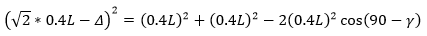(4)

Considering only small displacements (cos(90) ≈ γ; Δ2 ≈ 0) the shear strain, γ, was defined as follows,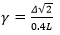(5)

Considering the diagonal compression test as a uniaxial compression with a loaded area of 0.4L and using Mohr’s circle, the shear stress τ (Pa) can be written as follows,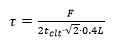(6)

where tclt was the total thickness of the CLT panel and F (N) is the force applied to the panel according to Fig. 3. This results in a fictitious shear modulus,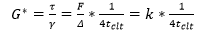(7)

where k is the stiffness F/Δ (N/m).

Because the loading setup for the diagonal compressed FE simulations not only resulted in shear but also compression and bending, a correction of the fictitious G* value was needed. The corrected G-value was described as follows,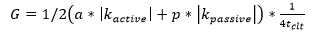(8)

where |kactive| and |kpassive| were the stiffnesses (N/m) in the active and passive directions, respectively.

The least squares method was used to determine constants a and p in Eq. 8. It gives a best-fit solution by minimizing the sum of the squares of the residuals between the G-values calculated for the pure shear models and the G-values for the corresponding FE simulated panel in diagonal compression.

Equation 3 was used to calculate the modulus of shear, G, for FE simulated panels in pure shear for the eight cases presented in Table 2. Equation 8 was used for the corresponding FE simulated panels in diagonal compression. Values of a and p both close to 1, would indicate that the FE simulated diagonal compression test resembled a pure shear case.

Diagonal Compression Tests

To verify the simulations, a total of 30 CLT panels were tested, 17 3-layer panels and 13 5-layer panels. The simulated CLT models verified by practical testing were 3, 3Ho, 5 and 5Ho, as outlined in Table 3. 14 of the 3-layer panels and 11 of the 5-layer panels had holes drilled in the perimeter (Fig. 3) and corner cut-outs made by a CNC machine, since they already had been used in a picture frame test to measure the shear modulus. The holes and cut-outs were not intended for this study. Three 3-layer panels and two 5-layer panels, reference panels, without holes and corner cut-outs (3T and 5T) were tested in order to check the impact of the holes and cut-outs on the results.

All panels, manufactured by a commercial CLT producer, were made out of Norway spruce (Picea abies) boards with the mean moisture contents of 7.4% and 8.6% for the 3- and 5-layer panels, respectively. The boards were classified as C24, according to SS-EN 338 (2016), which have a mean density of 420 kg/m3. They were glued on the flat side only, using polyurethane glue (i.e. no edge-gluing). To determine and verify if the boards were, in mean value, of class C24, the modulus of elasticity was measured according to SS-EN 408:2010+A1:2012 (2012) using clear wood samples obtained from the tested panels. The board dimensions and modulus of elasticity (E-modulus) for the tested panels are shown in Table 4.

Table 4. Material Properties of the Tested CLT Panels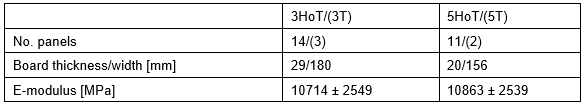“Ho” indicates panel with holes and cut-outs. “T” indicates tested. 3T and 5T also denoted reference panels.

The diagonal compression test was performed by compressing the CLT-panel in the diagonal direction, i.e., compressing two opposed corners using a steel crotch (see Fig. 3). Displacement sensors in the active (parallel to the force) and passive (orthogonal to the force) directions of the panels were separately measured, as shown in Fig. 4. Displacement sensors were put on both sides (front and back side) of the CLT panels to prevent the unintended influence of out of plane bending. Only mean values for the sensors on the front and back side, in the active and passive direction, of the CLT panel were used in the analysis.

The 3-layer panels were tested in three load cycles, from 0 to 50 kN, 0 to 100 kN, and finally until rupture (ultimate load). The 5-layer panels were also cycled three times, 0 to 100 kN, 0 to 150 kN, and 0 to rupture. The load was applied as increasing load cycles because of the uncertainty of the panel strength but also to make the panels settle. The load was applied at a 5 mm/min rate using a hydraulic displacement-controlled cylinder and the load level was measured using an Omegadyne LC412-100K load sensor (Sunbury, OH, USA). Displacement sensors were model Vishay HS25 (Malvern, PA, USA). An illustration of the force versus displacement curves from the diagonal compression can be seen in Fig. 5.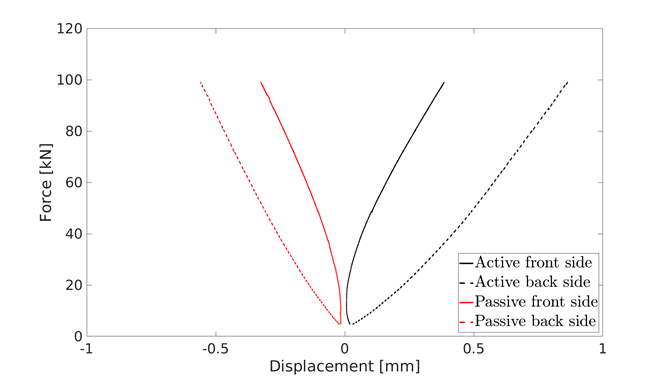Fig. 5. Illustration of the force versus displacement curves obtained from the four displacement transducers mounted on the CLT-panel

Two stiffness values, |k-active| and |k-passive|, were calculated using the mean value of the slope of the load-displacement curve. This was done during the second and third load cycle at a load between 60 kN and 80 kN. Stiffness k was defined as the force, F, divided by displacement, Δ. Displacement, Δ, was taken as either the displacement in the force direction (vertical direction in Fig. 4), in which case the stiffness was denoted active stiffness, or the horizontal direction, in which case the stiffness was called the passive stiffness (horizontal in Fig. 4). The shear modulus, G, was calculated according to Eq. 8 with constants a and p based on the results of the FE models.

RESULTS AND DISCUSSION

The shear modulus, Gpure, for the FE models in pure shear, is shown in Table 5. The expected shear modulus for the isotropic edge-glued models in pure shear, based on material data, was 120 MPa. Table 5 shows that the isotropic edge glued pure shear models produced a shear modulus of 119.7 MPa, which is equal to the expected result. The non-edge glued isotropic pure shear panels show lower values of the shear modulus than edge glued. The non-edge glued 3-layer isotropic CLT panel had a lower shear modulus than the corresponding 5-layer panel. The difference between the modulus of shear for the 3EgPs and 5EgPs models was due to different E-moduli.

Table 5. Shear Modulus, Gpure, for the FE Models in Pure Shear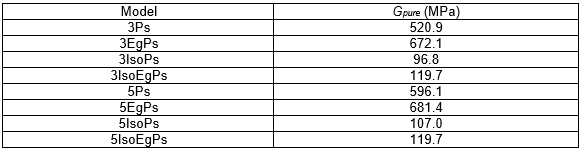Least Square Regression

The 8 FE simulated Gpure-values in Table 5 were matched to corresponding FE simulated diagonal compressed panel, and the least squares method yielded the values = 1.3 and p = 0.8. Table 6 shows the shear modulus for the FE simulated diagonal compressed models calculated from Eq.8 and the error compared to the result from the corresponding pure shear model presented in Table 6, which shows that the largest error was for the 3-layer panels. The 3-layer panel had an error of 4.1%, the other 3-layer models had errors around ±2.8%. The 5-layer panels had an error in the interval -3% to 2%. The mean absolute error for all models was 2.4%. A possible reason for the higher error in the 3-layer panel is that it is more susceptible to variation in the material and board thicknesses. This may give a higher error than the more homogenous 5-layer CLT-panel.

Table 6. Shear Modulus G Calculated from Eq. 8 and Error, Compared to Gpure, for Calibrated Finite Element Models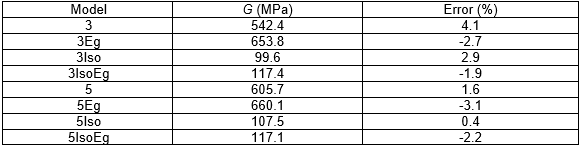Validation of Diagonal Compression Stiffness Values from FE Simulations vs. Tests

The stiffness values obtained from FE simulations of diagonal compression and corresponding practical tests were compared. The average measured stiffness values for all diagonally compressed test samples are listed in Table 7, which shows the stiffness based separately on active and passive directions. A lower stiffness, k, in the active direction was found for all tested panels, which means that the panels were compressed more in the active direction than stretched in the passive direction. Coefficients of variation (CV) for all four types of tested panels shown in Table 7 were almost equal for both the active and passive directed sensors. The stiffness was 32% higher in the passive direction than the active direction for both the 3- and 5-layer panels. The 3-layer panels had a CV value of 16%, which was higher than for 5-layer panels. This means that there was more variation between the 3-layer panels than between the 5-layer panels. The holes in the panels reduced the stiffness for both the 5- and 3-layer panels. The reference panels without holes had a lower CV than the panels with holes and cut-outs, which is due to the low number of tested reference panels.

Table 7. Average Stiffness, k, and Coefficient of Variation, CV, for the Diagonally Compressed Samples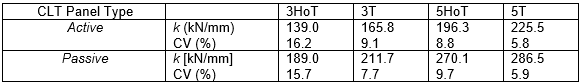“Ho” indicates models with holes and cut-outs. “T” indicates tested.

Stiffness from the FE-models can be seen in Table 8, together with comparisons with tested stiffness for models where test data exists. Total stiffness from FE-models and tests agree within a few per cent for most cases. The largest discrepancy was for the 5-layer panels with holes and cut-outs, where the FE-model had a 9.1% lower stiffness in the passive direction.

A reason for the higher stiffness in the practical tests than in FE simulations may be that panels in the tests were partly edge glued due to leakage of glue from flat sides into edge sides between the boards. The FE-model predicts 3% to 5% higher stiffness for the 3-layer panels with holes. A higher stiffness was also estimated by the FE-model for 3-layer panels without holes in the active direction. A reason for this is the large variation (CV value of 15%). For all panel types, the difference between the FE simulated and the tested active and passive stiffness values were similar except for the 5-layer panels with holes, where the differences were -4.4% and -9.1%, respectively.

In Table 8 panels with holes and cut-outs are compared with panels without. The results show that the mean stiffness in the active and passive direction decreased by 8.5% for panels with holes for both 3-and 5-layer panels. This is a quite large decrease considering that the holes only constitute about 5.1% of the total area of the panel. A possible reason could be that the outer part of the panel takes up a larger amount of the shear compared to the inner parts of the panel.

Table 8. Stiffness in the Active and Passive Direction for the Simulated FE Models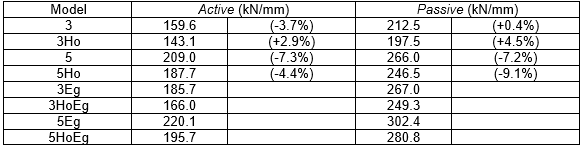In parenthesis, the difference relative to measured stiffness (Table 7). 3 and 5 indicates 3 and 5-layer CLT, respectively. “EG” indicates edge glued and “Ho” indicates with holes and cut-outs.

Shear Modulus Calculated from Diagonal Compression Tests

Table 9 shows the calculated shear modulus using diagonal compression test data.

Table 9. Shear Modulus, G, Calculated from Diagonal Compression Tests Using Eq. 8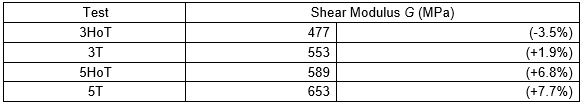3 and 5 indicates 3 and 5-layer CLT, respectively. The difference relative to FE calculated shear modulus, G, during diagonal compression is in parenthesis. “Ho” indicates models with holes and cut-outs. “T” indicates practical tested

Equation 8 was used to calculate the least squares constants a = 1.3 and p = 0.8. The results showed that the 5-layer panels without holes had a shear modulus of 653 MPa and the 3-layer panel 553 MPa. Compared to the FE calculated shear modulus for 5Eg (660 MPa), it seems that the tested 5-layer panels were almost edge glued, but 5T also has few tested panels. The holes and cut-outs in the panels decreased the modulus of shear by 14% and 10% for the 3- and 5-layer panel, respectively. This is in parity with the stiffness results and indicates that the influence of holes, in the perimeter, on modulus of shear was relatively larger compared to the removed area.

The shear modulus difference in the literature is dependent on panel set-up; in Brandner et al. (2017) a similar 3-layer panel (A2), was tested to have between 460 and 490 MPa. However, the board width used was 160 mm, 20 mm smaller than the ones used in this investigation. In Dujic et al. (2007) a 3-layer panel was measured to have a shear modulus of 500 to 700 MPa.

CONCLUSIONS

1. An equation for calculating the shear modulus from diagonal compression tests was obtained using FE calibration models.
2. The equation resulted in a shear modulus G of 653 MPa and 553 MPa for the practical tested 5- and 3-layer panels, without holes and cut-outs (named 5T and 3T), respectively.
3. The higher shear modulus G for the 5T panels was a result of partly edge glued boards and a low number of tested panels. Based on the FE model calibrations, a 606 MPa shear modulus was expected for 5T.
4. It has been shown that using the calibrated equation for shear modulus, realistic values can be attained from the diagonal compression test.
5. Panels with holes at the perimeter show a higher degree of loss of shear stiffness than expected considering the limited loss of material.

ACKNOWLEDGEMENTS

The experimental campaign presented in this paper is part of the EU-funded COST Action FP1402 working group and part of the Norwegian project “Increased use of wood in urban areas – WOOD/BE/BETTER”, funded by The Norwegian Research Council through the BIONÆR/BIONAER research program.

The authors at Luleå University of Technology were financed mainly by the European Union Interreg Nord programme in a research and innovation project called “Future CLT” from 2015–2018. The authors are grateful for their support.

REFERENCES CITED

Andreolli, M., Rigamonti, M. A., and Tomasi, R. (2014). “Diagonal compression test on cross laminated timber panels,” in: Proceedings of the 13th World Conference on Timber Engineering (WCTE 2014), Quebec.

BjØrnfot, A., Boggian, F., Steinsvik Nygård, A., and Tomasi, R. (2017). “Strengthening of traditional buildings with slim panels of cross-laminated timber (CLT),” in: Proceedings of the 4th International Conference on Structural Health Assessment of Timber Structures (SHATIS’17), Istanbul.

Brandner, R., Dietsch, P., Dröscher, J., Schulte-Wrede, M., Kreuzinger, H., and Sieder, M. (2017). “Cross laminated timber (CLT) diaphragms under shear: Test configuration, properties and design,” Construction and Building Materials 147, 312-327. DOI: 10.1016/j.conbuildmat.2017.04.153

Brandner, R., Flatscher, G., Ringhofer, A., Schickhofer, G., and Thiel, A. (2016). “Cross laminated timber (CLT) overview and development,” European Journal of Wood and Wood Products 74(3), 331-351. DOI: 10.1007/s00107-015-0999-5

Brandner, R., Tomasi, R., Moosbrugger, T., Serrano, E., and Dietsch, P. (eds.). (2018). Properties, Testing and Design of Cross Laminated Timber: A State-of-the-art Report by COST Action FP1402/WG 2, Shaker Verlag, Aachen, Germany.

Brandner, R., Dietsch, P., Dröscher, J., Schulte-Wrede, M., Kreuzinger, H., Sieder, M., Schickhofer, G., and Winter, S. (2015). “Shear properties of cross laminated timber (CLT) under in‐plane load: Test configuration and experimental study,” in: International Network on Timber Engineering Research (INTER) 2015, Sibenik, Croatia, 24-27 August 2015.

Colman, A. G., Bridgens, B. N., Gosling, P. D., Jou, G. T., and Hsu, X.Y. (2014). “Shear behavior of architectural fabrics subjected to biaxial tensile loads,” Composites Part A: Applied Science and Manufacturing 66, 163-174. DOI: 10.1016/j.compositesa.2014.07.015

Dujic, B., Klobcar, S., and Zarnic, R. (2007). “Influence of openings on shear capacity of wooden walls,” in: Proceedings of International Council for Research and Innovation in Building and Construction (Working Commission W18 – Timber structures (CIB-W18/40-15-6)), Bled, Slovenia.

Franzoni, L., Lebee, A., Lyon, F., and Foret, G. (2017). “Elastic behavior of cross laminated timber and timber panels with regular gaps,” Engineering Structures 141, 402-416. DOI: 10.1016/j.engstruct.2017.03.010

Frocht, M. M. (1931). “Recent advances in photoelasticity and an investigation of the stress distribution in square blocks subjected to diagonal compression,” Transactions ASME 55, 135-153.

Kreuzinger, H. and Sieder, M. (2013). “Einfaches Prufverfahren zur Bewertung der Schubfestigkeit von Kreuzlagenholz/Brettsperrholz,” Bautechnik 90(5), 314-316. DOI: 10.1002/bate.201300024 (in German)

Li, L., Zhao, Y., Vuong, H., Chen, Y., Yang, J., and Duan, Y. (2014). “In-plane shear investigation of biaxial carbon non-crimp fabrics with experimental test and finite element modeling,” Materials and Design 63, 757-765. DOI: 10.1016/j.matdes.2014.07.007

Lukacs, I., Björnfot, A., and Tomasi, R. (2019). “Strength and stiffness of cross-laminated timber (CLT) shear walls: State-of-the-art of analytical approaches,” Engineering Structures 178(1), 136-147. DOI: 10.1016/j.engstruct.2018.05.126

SS-EN 1995-2 (2004). Eurocode 5: “Design of timber structures – Part 2: Bridges,” Swedish Standard Institute, Stockholm, Sweden.

SS-EN 338 (2016). “Structural timber – Strength classes,” Swedish Standard Institute, Stockholm, Sweden.

SS-EN 408:2010+A1:2012 (2012). “Timber structures – Structural timber and glued laminated timber – Determination of some physical and mechanical properties,” Swedish Standard Institute, Stockholm, Sweden.

Article submitted: February 25, 2019; Peer review completed: May 3, 2019; Revised version received and accepted: May 23, 2019; Published: May 29, 2019.

DOI: 10.15376/biores.14.3.5559-5572